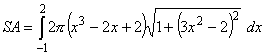## MAT 162

1.      Evaluate the following integrals.

1.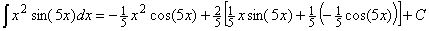2.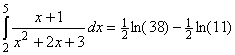3.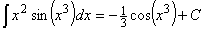4.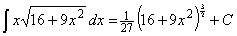5.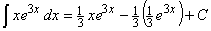6.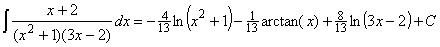7.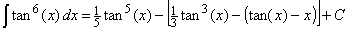8.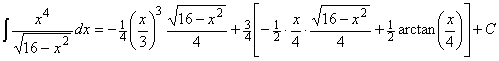9.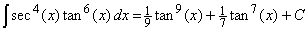10.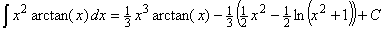11.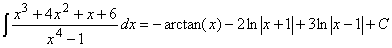12.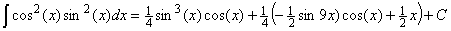13.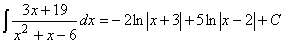14.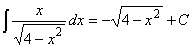15.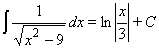16.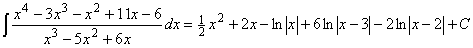17.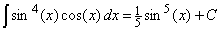18.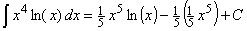19.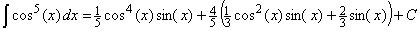20.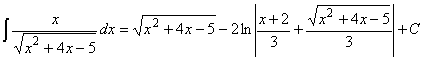21.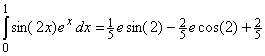22.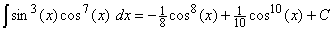2.      Set up the integral to find the length of the curve.  (You do not need to simplify or evaluate the integral.)  (5 points)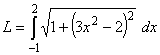3.      Set up the integral to find the volume of the solid generated by revolving the area under the curve around the x-axis.  (You do not need to simplify or evaluate the integral.)  (5 points)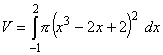4.      Set up the integral to find the surface area of the solid in Question #3.  (You do not need to simplify or evaluate the integral.)  (5 points)